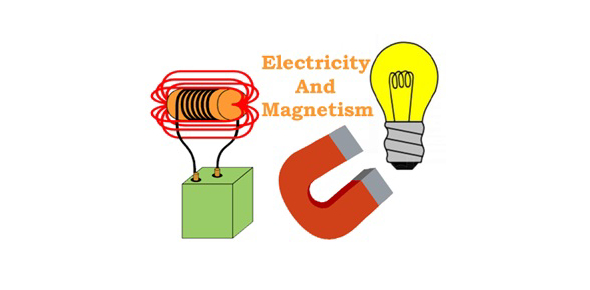# Trivia: Quiz On Electricity And Magnetism!

18 Questions | Total Attempts: 819SettingsHave you ever needed to understand more about electricity and magnetism? This quiz could help you. There is a definite relationship between electricity and magnetism. Magnetic fields are created by moving charges. Electricity is the existence of the motion of charged elements. Magnetism is the concept of interaction between moving objects. Magnetic fields can travel quite far. This fascinating quiz will attract a high score.

• 1.
A measure of a material's ability to retain magnetism.
• A.

Flux Density

• B.

Coercive Force

• C.

Flux Lines

• 2.
Magnetic strength is measured in flux density.
• A.

True

• B.

False

• 3.
Reluctance
• A.

Is resistance to magnetism.

• B.

Is a variable resistor used as a voltage divider.

• C.

Is a measure of a materials ability to become magnetized.

• 4.
Occurs when all the molecules of the magnetic materials are lined up
• A.

Ampere-Turns

• B.

Reluctance

• C.

Saturation

• 5.
Ampere-Turns are determined by multiplying the number of turns of wire by the current flow.
• A.

True

• B.

False

• 6.
The amount of magnetism left in a material after the magnetizing force has stopped.
• A.

Residual Magnetism

• B.

Potentiometer

• C.

Saturation

• 7.
Permeability is a measure of a materials ability to become demagnetized
• A.

True

• B.

False

• 8.
Flux Lines
• A.

Magnetic lines of force are called flux

• B.

Magnetic lines of flux repel each other and never cross

• C.

They do not flow but are always assumed to be in the North and South directions

• D.

All the above

• 9.
Natural Magnetic is called
• A.

Lodestones

• B.

• C.

• D.

All the above

• 10.
Resistors:
• A.

Limit the flow of current in a circuit and are voltage dividers

• B.

Are voltage dividers and have high power ratings

• C.

Have higher power ratings and limit current flow

• D.

All the above

• 11.
Have higher power ratings, should be mounted vertically.
• A.

Metal film resistors

• B.

Carbon film resistors

• C.

Wire-wound Resistors

• 12.
A potentiometer is a variable resistor used as a voltage divider.
• A.

True

• B.

False

• 13.
Metal Film Resistor:
• A.

Have a higher tolerance

• B.

Must be mounted vertically

• C.

Don’t change value with age

• D.

All the above

• 14.
Demagnetizing occurs by:
• A.

Being struck

• B.

Holding another magnetic close

• C.

Cooling

• D.

An AC unit with a power connection

• E.

Heating

• F.

Smashing with a sledge-hammer

• G.

A strong electromagnetic connected to an AC line

• 15.
Left-handed Rule if the direction of current flow is reversed, the polarity of the magnetic field is not reversed.
• A.

True

• B.

False

• 16.
Energy is required to create a magnetic field, but no energy is required to maintain a magnetic field.
• A.

Permanent Magnetics

• B.

Residual Magnetism

• 17.
Magnetic Materials:
• A.

Ferromagnetic Materials – easily magnetized

• B.

Paramagnetic Materials – can be magnetized but not as easily.

• C.

Diamagnetic Materials – cannot be magnetized.

• D.

All the above

• 18.
Basic Law of Magnetism unlike poles _______ and like poles ________.
• A.

Repel and Attract

• B.

Repel and Repel

• C.

Attract and Repel

• D.

Attract and Attract

Related TopicsBack to top